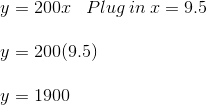# Representations of Functions: Function Tables, Graphs & Equations

Lesson Transcript
Instructor: Laura Pennington

Laura received her Master's degree in Pure Mathematics from Michigan State University, and her Bachelor's degree in Mathematics from Grand Valley State University. She has 20 years of experience teaching collegiate mathematics at various institutions.

Functions show up in our daily lives more often than we realize. In this lesson, we will go over the different ways of representing a function using function tables, equations, and graphs. Updated: 10/20/2020

## Functions

Most of us have worked a job at some point in our lives, and we do so to make money. Consider a job where you get paid $200 a day. If you only work a fraction of the day, you get that fraction of$200. Thus, the total amount of money you make at that job is determined by the number of days you work.

Mathematically speaking, this scenario is an example of a function. A function is a relationship between two variables, such that one variable is determined by the other variable. In our example, if we let x = the number of days we work and y = the total amount of money we make at this job, then y is determined by x, and we say y is a function of x.

We've described this job example of a function in words. There are other ways to represent a function, as well. We're going to look at representing a function with a function table, an equation, and a graph. Let's get started!

An error occurred trying to load this video.

Try refreshing the page, or contact customer support.

Coming up next: Scatterplots and Line Graphs: Definitions and Uses

### You're on a roll. Keep up the good work!

Replay
Your next lesson will play in 10 seconds
• 0:04 Functions
• 0:59 Function Table
• 2:16 Function Equation
• 3:08 Function Graphs
• 4:14 Lesson Summary
Save Save

Want to watch this again later?

Timeline
Autoplay
Autoplay
Speed Speed

## Function Table

A function table is a table of ordered pairs that follows the relationship, or rule, of a function. To create a function table for our example, let's first figure out the rule that defines our function. We have that each fraction of a day worked gives us that fraction of $200. Thus, if we work one day, we get$200, because 1 * 200 = 200. If we work two days, we get $400, because 2 * 200 = 400. If we work 1.5 days, we get$300, because 1.5 * 200 = 300. Are we seeing a pattern here?

To find the total amount of money made at this job, we multiply the number of days we have worked by 200. Thus, our rule is that we take a value of x (the number of days worked), and we multiply it by 200 to get y (the total amount of money made).

A function table can be used to display this rule. As you can see here, in the first row of the function table, we list values of x, and in the second row of the table, we list the corresponding values of y according to the function rule.

 x = # days worked 1 2 3 3.5 5 7.25 8 y = total money made 200 400 600 700 1000 1450 1600

Sometimes function tables are displayed using columns instead of rows. When this is the case, the first column displays x-values, and the second column displays y-values.

 x = # days worked y = total money made 1 200 2 400 3 600 3.5 700 5 1000 7.25 1450 8 1600

## Function Equation

Another way to represent a function is using an equation. In this representation, we basically just put our rule into equation form. For our example, the rule is that we take the number of days worked, x, and multiply it by 200 to get the total amount of money made, y. Putting this in algebraic terms, we have that 200 times x is equal to y. In equation form, we have y = 200x.

Once we have our equation that represents our function, we can use it to find y for different values of x by plugging values of x into the equation. For example, if we wanted to know how much money you would make if you worked 9.5 days, we would plug x = 9.5 into our equation.We see that if you worked 9.5 days, you would make \$1,900. Not bad!

To unlock this lesson you must be a Study.com Member.

### Register to view this lesson

Are you a student or a teacher?